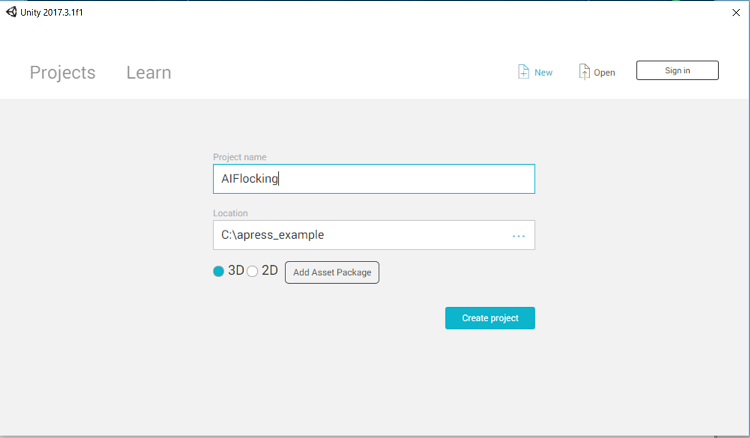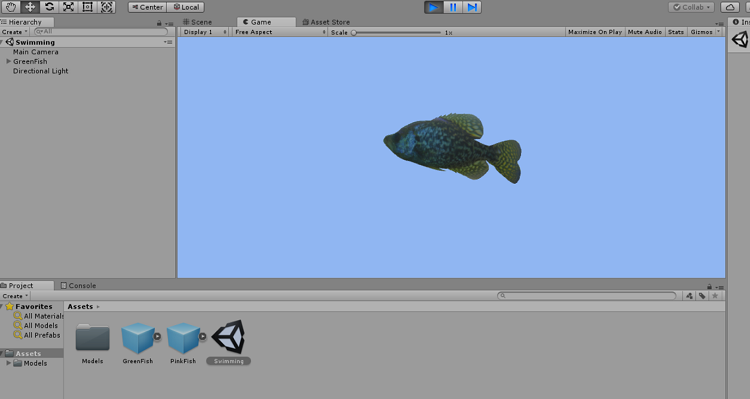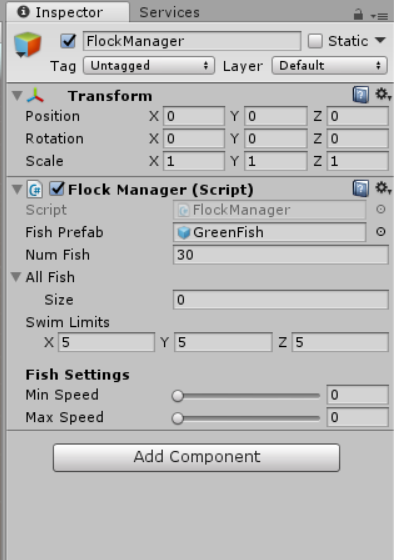# Fish Flocking with Unity*: Simulating the Behavior of Object Moving with AI

ID 671603
Updated 1/21/2019
Version Latest
PublicBy

## Introduction

This article describes how flocking behavior is used to resemble an AI behavior, and implement that behavior on a group of fish. The step-by-step process for this important AI characteristic includes:

1. Start working with Unity* integration development environment (IDE)
2. Use the fish asset to start building the environment
3. Replicate the flocking behavior

## System Requirements

• Standard ASUS ROG* Laptop
• 4th generation Intel® Core™ i7 processor
• 8GB RAM
• Windows* 10 Enterprise edition

## Flocking

Flocking is a behavior in which objects move or work together as a group. The behavior has depth and parallels to shoaling and schooling behavior in fish, and to the swarming behavior of insects and herds of land animals. The flocking simulation is implemented as an AI logic simulation to keep the objects___together to generate the crowd______. This involves separation, alignment and cohesion as explained in Crowd Simulation as a Flocking Behavior and Windows* Mixed Reality: Part 1.

## Creating the Project

To use Unity IDE to open up the AI fish package, begin by creating a new project.Figure 1. Create a new project in Unity*

Then import the package: Assets > Import Package > Custom PackageFigure 2. Import package in Unity

Import the entire Fish package.Figure 3. Import the entire fish package

View the scene with Asset package.Figure 4. View the scene with Asset package

Start working with the AI behavior patterns by opening the asset in Unity*, where a pink fish and a green fish are shown. When the swimming scene is opened in Unity, the green fish has a swimming animation attached to it.

To implement flocking, more fish need to be created since flocking is a group activity. Create a flocking manager by right-clicking in the hierarchy to create empty object, and rename as FlockManager.

To begin the coding process, create different c# scripts for the functionality. The first C# script to create and attach will be the FlockManager.

The script as generated looks like this:

using System.Collections;
using System.Collections.Generic;
using UnityEngine;

public class FlockManager : MonoBehaviour {

// Use this for initialization
void Start () {

}

// Update is called once per frame
void Update () {

}
}

Add a piece of code to the FlockManager to create an array of fish object instantiations and put them in random locations around the flock manager.

First create a public variable named fishPrefab:

public GameObject fishPrefab;

The fishPrefab can be a green fish or pink fish. Then set public numfish to 20. This can be changed accordingly:

public int numFish = 20;

Change the number of fish through the inspector windows in Unity IDE. Then create an array of allFish. As they are instantiated, they’ll go, that is the number of fishes will go inside the allFish array:

public GameObject[] allFish;

Establishing swim limits sets the range of where the fish can be, acting as a box around the flock manager. Use the swim limits to generate the location of the fish in the box. The logic will happen inside the start function:

public Vector3 swimLimits = new Vector3(5,5,5);

First, create all of the fish. Make the array big enough to hold them.

allFish = new GameObject[numFish];

There is a for loop that will cycle through a set number of times. Position the fish:

Vector3 pos;

This position is based on the position of the flock manager plus a random Vector3 limit around the swim limits between negative swim limits and positive swim limits. If using a larger box, alter it as shown below:

public Vector3 swimLimits = Vector3 (5,5,5);

Changed the parameters to:

public Vector3 swimLimits = Vector3 (10, 10,10);

Figure 5. Parameters to set the position for a larger array box

The updated position looks like this:

Vector3 pos = this.transform.position + new Vector3(Random.Range(-swimLimits.x,swimLimits.x),

Random.Range(-swimLimits.y,swimLimits.y),

Random.Range(-swimLimits.z,swimLimits.z));

The fish are in a position which is instantiated from prefab at the position calculated using a neutral rotation. Then will fill up the array:

allFish[i] = (GameObject) Instantiate(fishPrefab, pos, Quaternion.identity);

The update, as of now, adding all parameters and declaring the variables, looks like this:

using System.Collections;
using System.Collections.Generic;
using UnityEngine;

public class FlockManager : MonoBehaviour {
public GameObject fishPrefab;
public int numFish = 20;
public GameObject[] allFish;
public Vector3 swimLimits = new Vector3(5,5,5);

// Use this for initialization
void Start () {
allFish = new GameObject[numFish];
for(int i = 0; i < numFish; i++)
{
Vector3 pos = this.transform.position + new Vector3(Random.Range(-swimLimits.x,swimLimits.x),
Random.Range(-swimLimits.y,swimLimits.y),
Random.Range(-swimLimits.z,swimLimits.z));
allFish[i] = (GameObject) Instantiate(fishPrefab, pos, Quaternion.identity);
//allFish[i].GetComponent<Flock>().myManager = this;
}

}

// Update is called once per frame
void Update () {

}
}

Now add back the code and the parameters into Unity and run it. First, add the FlockManager script to the empty FlockManager object created. Delete the greenfish prefab. In the FlockManager add up the fish prefab.Figure 5. FlockManager parameters

Now run the scene.Figure 6. Running the scene in Unity

The fish all appear in the hierarchy and within the inspector window; they are within the allFish array so that each fish can find every other fish in the flock.Figure 7. View of the allFish array

## Fish Movement

Now to get the fish moving, begin by adding some speed values to the fish, using the FlockManager to set different variables for particular fish in each flock; building multiple flocks for the fish.

Use the FlockManager to add public values, putting up a range that acts as a slider. First, make header settings and declare two variables: public minspeed and public maxspeed.

[Header("Fish Settings")]
[Range(0.0f, 5.0f)]
public float minSpeed;
[Range(0.0f, 5.0f)]
public float maxSpeed;


The changes are reflected in the inspector window:Figure 8. FlockManager settings in the inspector window

Create a new c# file for the fish and call it Flock. Add the script to both greenfish and pinkfish.

Now let’s open the default script:

using System.Collections;
using System.Collections.Generic;
using UnityEngine;

public class Flock : MonoBehaviour {

// Use this for initialization
void Start () {

}

// Update is called once per frame
void Update () {

}
}

The first thing needed is a link back to the flock manager:

public FlockManager myManager;

Then float speed is needed:

float speed;

In the start method, declare the speed to Random.range from manager’s minimum speed settings to the maximum speed settings.

speed = Random.Range(myManager.minSpeed,myManager.maxSpeed);

In the update method, declare the translate statement that will move the fish forward or along the z axis. The value of the speed of the fish goes inside the z axis.

transform.Translate(0, 0, Time.deltaTime * speed);

To get the connection between the FlockManager.cs script and the Flock.cs script inside the for loop of FlockManager.cs just after the fishes are declared:

allFish[i].GetComponent<Flock>().myManager = this;

The updated code looks like this:

using System.Collections;
using System.Collections.Generic;
using UnityEngine;

public class FlockManager : MonoBehaviour {
public GameObject fishPrefab;
public int numFish = 20;
public GameObject[] allFish;
public Vector3 swimLimits = new Vector3(5,5,5);

[Range(0.0f, 5.0f)]
public float minSpeed;
[Range(0.0f, 5.0f)]
public float maxSpeed;

// Use this for initialization
void Start () {
allFish = new GameObject[numFish];
for(int i = 0; i < numFish; i++)
{
Vector3 pos = this.transform.position + new Vector3(Random.Range(-swimLimits.x,swimLimits.x),
Random.Range(-swimLimits.y,swimLimits.y),
Random.Range(-swimLimits.z,swimLimits.z));
allFish[i] = (GameObject) Instantiate(fishPrefab, pos, Quaternion.identity);
allFish[i].GetComponent<Flock>().myManager = this;
}

}

// Update is called once per frame
void Update () {

}
}

Save the code and see the result in the scene.Figure 9. View the resulting scene

## Flocking Rules

The basic rules when flocking fish are:

#### Move toward the average position of the group

(which is the sum of all positions/number in the group). Taking all the individual members’ positions, adding them together, and dividing by the number in the group helps determine where individuals are located and how they have turned toward the group.

#### Align with the average heading of the group

Add the headings of all individual fish and divide by the size of the group; this produces an average vector to use in aligning this movement.

#### Avoid crowding other group members

To avoid neighbors, individuals must be aware of their neighbors’ position and when to turn away from them. To calculate a new heading, individuals add up a vector for moving toward the center of the group, a vector to align with the group’s heading, and a vector to turn away from the neighbor.

## The Flocking Effect and the Flocking Rules

Certain values in the FlockManager need to be set for the behavior of the fish, including:

[Range(1.0f, 10.0f)]
public float neighbourDistance;
[Range(0.0f, 5.0f)]
public float rotationSpeed;

Neighbor distance is turned down to check that there is no more flocking. When neighbor distance is turned back up, it doesn’t bring all the fish together. Rotation speed will also affect this. When the rotation speed is increased, some emergent behavior will start to be seen.

Return to the code and make some changes. To bring in the flocking effect, go to the flocking.cs code and write a new method ApplyRules() in the update method.

Void ApplyRules()

First this creates a holder gos that holds all of the fish in the current flock.

GameObject[] gos;
gos = myManager.allFish;

Calculate the average center, which will be stored in vcenter. Next, calculate the average avoidance vector as well, which is vavoid. Calculate the avoidance vector, which is set to 0.

Vector3 vcentre = Vector3.zero;
Vector3 vavoid = Vector3.zero;

The float gspeed is the global speed of the group – the speed at which the average group is moving and used in the calculation.

float gSpeed = 0.01f;

Next is the float nDistance. We ask each fish how far they are then work to find that they are closer to the group.

float nDistance;

A groupsize counter will count the number of fish that are in the group, acting like a smaller subsection of the flock in the neighbor distance.

int groupSize = 0;

All will be initialized to zero.

Loop through all of the fish in the flock, using a fish for each loop. The structure of the fishes organized within the for each loop.

foreach (GameObject go in gos)

{

}

The current fish go is not equal to the fish that are running in the code (if(go != this.gameObject) in this instance so complete the following process to work around the distance to the neighbor. Find the distance Vector3.distance between the game object in the arrays position (go.transform.position) and the position in the world (this.transform.position).

nDistance = Vector3.Distance(go.transform.position,this.transform.position);

Next determine if the distance is less than or equal to the neighbor distance, which makes them a fish of interest, one that will be considered part of the closenet group. Add that fish’s position to the center that is vcenter. Vcenter will be the average position of the group. Loop around and add all the positions together and divide by group size. At the same time, increase the group size by 1. After the loop has gone through, calculate the average.

if(nDistance <= myManager.neighbourDistance)
{
vcentre += go.transform.position;
groupSize++;
if(nDistance < 1.0f)
{
vavoid = vavoid + (this.transform.position - go.transform.position);
}

}

Next use an if statement to test if the nDistance is less than a much smaller value, which has been hard coded here as 1.0. This defines how close a fish can be to another fish before it might act to avoid it. If the nDistance is less than 1.0 f, then the average vector should be the vector itself plus the vector away from the fish (that is, the current position minus the position to get away from).

                                                                  if(nDistance < 1.0f)
{
vavoid = vavoid + (this.transform.position - go.transform.position);
}

Global speed or gspeed is adding the total speed of a particular fish in the flock. Attach the flock code that is attached to a given fish, then grab its speed and attach it to its gspeed.

Flock anotherFlock = go.GetComponent<Flock>();
gSpeed = gSpeed + anotherFlock.speed;

The entire flow for each statement code is summarized in one place:

foreach (GameObject go in gos)
{
if(go != this.gameObject)
{
nDistance = Vector3.Distance(go.transform.position,this.transform.position);
if(nDistance <= myManager.neighbourDistance)
{
vcentre += go.transform.position;
groupSize++;

if(nDistance < 1.0f)
{
vavoid = vavoid + (this.transform.position - go.transform.position);
}

Flock anotherFlock = go.GetComponent<Flock>();
gSpeed = gSpeed + anotherFlock.speed;
}
}
}

By the time each loop is completed through every fish in the flock and examined whether it’s the closest neighbor, its position and avoidance vector are found.

Underneath the logic written within for each loop, is an if statement that asks if the group size is greater than zero. If the fish are moving along and no other fish are around it, and the group size becomes zero, the rule will not apply and the fish will keep moving in a straight line. If this fish is influenced by any other fish or another group, then find the average center, the vcentre vector that were calculated. Add them all together and divide by the group size.

Vcentre = vcentre/groupSize

Speed itself of the fish is said to be gSpeed divided by groupSize

Speed = gSpeed/groupSize;

With that information, the direction the fish needs to travel or the direction in which the fish wants to travel can be determined. The Vector3 direction is:

Vector3 direction =(vcentre + vavoid) –transform.position

If(direction ==0) then we are facing the right direction

And

If(direction !=Vector3.zero)

Next is to use the slerp to rotate (transform.rotate) accordingly for the rotation speed. Slowly turn the fish in the required direction.

if(direction != Vector3.zero)
transform.rotation = Quaternion.Slerp(transform.rotation,
Quaternion.LookRotation(direction),
myManager.rotationSpeed * Time.deltaTime);

The entire updated code for flock.cs looks like this:

using System.Collections;
using System.Collections.Generic;
using UnityEngine;

public class Flock : MonoBehaviour {

public FlockManager myManager;
float speed;

// Use this for initialization
void Start () {
speed = Random.Range(myManager.minSpeed,
myManager.maxSpeed);

}

// Update is called once per frame
void Update () {
transform.Translate(0, 0, Time.deltaTime * speed);
ApplyRules();

}
void ApplyRules()
{
GameObject[] gos;
gos = myManager.allFish;

Vector3 vcentre = Vector3.zero;
Vector3 vavoid = Vector3.zero;
float gSpeed = 0.01f;
float nDistance;
int groupSize = 0;

foreach (GameObject go in gos)
{
if(go != this.gameObject)
{
nDistance = Vector3.Distance(go.transform.position,this.transform.position);
if(nDistance <= myManager.neighbourDistance)
{
vcentre += go.transform.position;
groupSize++;

if(nDistance < 1.0f)
{
vavoid = vavoid + (this.transform.position - go.transform.position);
}

Flock anotherFlock = go.GetComponent<Flock>();
gSpeed = gSpeed + anotherFlock.speed;
}
}
}

if(groupSize > 0)
{
vcentre = vcentre/groupSize;
speed = gSpeed/groupSize;

Vector3 direction = (vcentre + vavoid) - transform.position;
if(direction != Vector3.zero)
transform.rotation = Quaternion.Slerp(transform.rotation,
Quaternion.LookRotation(direction),
myManager.rotationSpeed * Time.deltaTime);

}
}
}

Now run the application in Unity to see the flock movement.Figure 10. View the resulting scene

## Conclusion

This article went step-by-step through the process used to create a flocking effect in fish; an emergent AI behavior using Unity. Flocking rules were applied to achieve the flock movement results. For more information on implementing emergent behavior as crowd simulation, visit Crowd Simulation as a Flocking Behavior and Windows* Mixed Reality: Part 1.